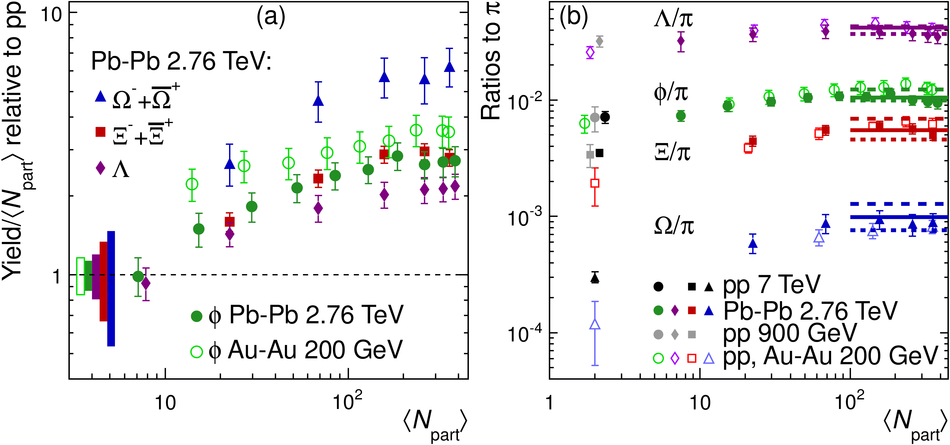# K*(892)$^{0}$ and $Φ$(1020) production in Pb-Pb collisions at $\sqrt{s_{\rm NN}}$ = 2.76 TeV

The yields of the K*(892)$^{0}$ and $\Phi$(1020) resonances are measured in Pb-Pb collisions at $\sqrt{s_{\rm NN}}$ = 2.76 TeV through their hadronic decays using the ALICE detector. The measurements are performed in multiple centrality intervals at mid-rapidity (|$y$|<0.5) in the transverse-momentum ranges 0.3 < $p_{\rm T}$ < 5 GeV/$c$ for the K*(892)$^{0}$ and 0.5 < $p_{\rm T}$ < 5 GeV/$c$ for the $\Phi$(1020). The yields of K*(892)$^{0}$ are suppressed in central Pb-Pb collisions with respect to pp and peripheral Pb-Pb collisions (perhaps due to rescattering of its decay products in the hadronic medium), while the longer lived $\Phi$(1020) meson is not suppressed. These particles are also used as probes to study the mechanisms of particle production. The shape of the $p_{\rm T}$ distribution of the $\Phi$(1020) meson, but not its yield, is reproduced fairly well by hydrodynamic models for central Pb-Pb collisions. In central Pb-Pb collisions at low and intermediate $p_{\rm T}$, the p/$\Phi$(1020) ratio is flat in $p_{\rm T}$, while the p/$\pi$ and $\Phi$(1020)/$\pi$ ratios show a pronounced increase and have similar shapes to each other. These results indicate that the shapes of the $p_{\rm T}$ distributions of these particles in central Pb-Pb collisions are determined predominantly by the particle masses and radial flow. Finally, $\Phi$(1020) production in Pb-Pb collisions is enhanced, with respect to the yield in pp collisions and the yield of charged pions, by an amount similar to the $\Lambda$ and $\Xi$.

Figures

## Figure 1

 Example invariant-mass distributions for the $\rm K^{*0}$ in the 0-20% centrality interval in two $\pt$ ranges: $0.8< \pt< 1.2$ GeV/$c$ [panels (a) and (b)] and $2.5< \pt< 3$ GeV/$c$ [panels (c) and (d)]. Panels (a) and (c) show the unlike-charge invariant-mass distributions for $\rm K^{*0}$ with combinatorial backgrounds. The normalized mixed-event combinatorial background is within 0.5% (0.7%) of the unlike-charge distribution for the low (high) $\pt$ bin over the invariant-mass range shown here. The statistical uncertainties are not visible given the vertical scale. Panels (b) and (d) show the invariant-mass distributions after subtraction of the mixed-event background (plotted with statistical uncertainties) with fits to describe the peaks of the $\rm K^{*0}$ (solid curves) and residual backgrounds (dashed curves). In the interval $0.8< \pt< 1.2$ GeV/$c$ ($2.5< \pt< 3$ GeV/$c$ ), the uncorrected $\rm K^{*0}$ yield is 7.4 (2.4) million, or 2.4 (0.80) per event; the signal-to-background ratio is $1.1\times10^{-4}$ ($5.6\times10^{-4}$) and the significance of the $\rm K^{*0}$ peak is 17 (25).## Figure 2

 Example invariant-mass distributions for the $\Phi$ in the 0-10% centrality interval in two $\pt$ ranges: $0.8< \pt< 1$ GeV/$c$ [panels (a) and (b)] and $2.5< \pt< 3$ GeV/$c$ [panels (c) and (d)]. Panels (a) and (c) show the unlike-charge invariant-mass distributions for $\Phi$ with combinatorial backgrounds. The normalized mixed-event combinatorial background is within 0.5% (1%) of the unlike-charge distribution for the low (high) $\pt$ bin over most of the invariant-mass range shown here (with the exception of the $\Phi$ peak itself and $m_{\rm{KK}} < 0.995$ GeV/$c^2$ ). The statistical uncertainties are not visible given the vertical scale. Panels (b) and (d) show the invariant-mass distributions after subtraction of the mixed-event background (plotted with statistical uncertainties) with fits to describe the peaks of the $\Phi$ (solid curves) and residual backgrounds (dashed curves). In the interval $0.8 < \pt < 1$ GeV/$c$ ($2.5< \pt < 3$ GeV/$c$ ), the uncorrected $\Phi$ yield is 174,000 (149,000), or 0.11 (0.095) per event; the signal-to-background ratio is 0.01 (0.0035) and the significance of the $\Phi$ peak is 38 (21).## Figure 3

 The product of the acceptance and the resonance reconstruction efficiency $A\times\varepsilon_{\rm rec}$ as a function of $\pt$ for $\rm K^{*0}$ (a) and $\Phi$ (b) mesons, calculated from simulated Pb-Pb collisions at $\snn$ = 2.76 TeV. For the $\Phi$ meson, only five examples for wide centrality intervals are shown. The acceptance $A$ includes the effect of the resonance pair rapidity cut ($|y|< 0.5$). The values shown here do not include the branching ratios. Only statistical uncertainties are shown.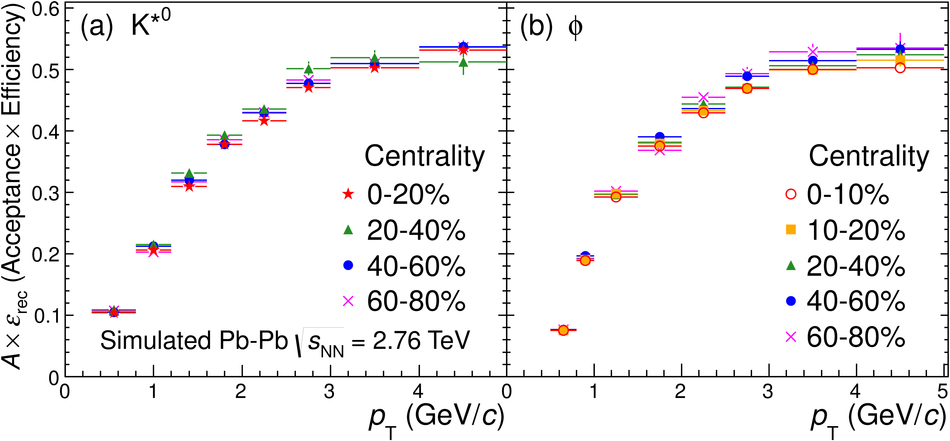## Figure 4

 Transverse-momentum distributions of $\rm K^{*0}$ (a) and $\Phi$ (b) mesons in multiple centrality intervals with blast-wave fitting functions. The data are the bin-averaged yields plotted at the bin centers. The statistical uncertainties are shown as bars and are frequently smaller than the symbol size. The total systematic uncertainties (including $\pt$-uncorrelated and $\pt$-correlated components) are shown as boxes.## Figure 5

 Measured $\rm K^{*0}$ meson mass (a) and width (b) in Pb--Pb collisions at $\snn$ = 2.76 TeV in the 0-20% and 60-80% centrality intervals, along with the values extracted from Monte-Carlo HIJING simulations. Measured $\Phi$ meson mass (c) and width (d) in Pb--Pb collisions at $\snn$ = 2.76 TeV in the 0-10% and 70-80% centrality intervals. The $\Phi$ width extracted from HIJING simulations is also shown. The vacuum values of the $\rm K^{*0}$ and $\Phi$ mass and width \cite{PDG} are indicated by the horizontal dashed lines. The statistical uncertainties are shown as bars and the total systematic uncertainties (including $\pt$-uncorrelated and $\pt$-correlated components) are shown as boxes.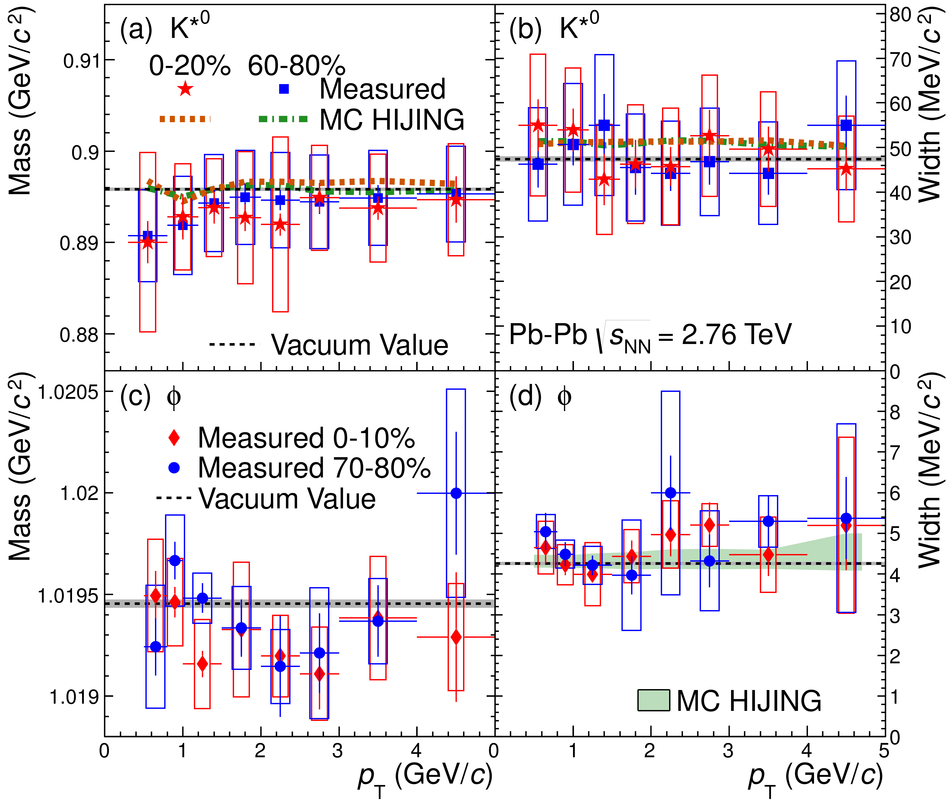## Figure 6

 Ratios of $\pt$-integrated particle yields $\rm K^{*0}/K^{-}$ and $\Phi/K^{-}$ as a function of $({\rm d} N_{\rm ch}/\rm d\eta)^{1/3}$ for Pb-Pb collisions at $\snn$ = 2.76 TeV and pp collisions at $\sqrt{s}$ = 7 TeV. The values of ${\rm d} N_{\rm ch}/\rm d\eta$ were measured at mid-rapidity. The statistical uncertainties are shown as bars. The shaded boxes show systematic uncertainties that are not correlated between centrality intervals, while the open boxes show the total systematic uncertainties including both correlated and uncorrelated sources. The values given by a grand-canonical thermal model with a chemical freeze-out temperature of 156 MeV are also shown \cite{Stachel_SQM2013}.## Figure 7

 Particle ratios $\rm K^{*0}/K^{-}$ [panels (a) and (b)] and $\Phi/K^{-}$ [panels (c) and (d)] in pp, d-Au, and A-A collisions. In panels (a) and (c) these ratios are presented for different centrality intervals as a function of $({\rm d} N_{\rm ch}/\rm d\eta)^{1/3}$. The values of ${\rm d} N_{\rm ch}/\rm d\eta$ were measured at mid-rapidity. In panels (b) and (d), these ratios are presented for pp, central d-Au, and central A-A collisions as a function of $\snn$. The values given by a grand-canonical thermal model with a chemical freeze-out temperature of 156 MeV are also shown. For quantities marked *", boxes represent the total uncertainty (separate uncertainties are not reported). Otherwise, bars represent the statistical uncertainties and boxes represent the systematic uncertainties (including centrality-uncorrelated and centrality-correlated components). For the d-Au data in panels (a) and (b), the numerator yield is derived from a combination of the charged and neutral $\mathrm{K}^{*}(892)$ states. In panel (c), the two most central $\Phi/K^{-}$ points for Au-Au collisions are for overlapping centrality intervals (0-5\% and 0-10\%). The following points have been shifted horizontally for visibility: the lowest-multiplicity d-Au points in panels (a) and (c), the d-Au points in panels (b) and (d), and the pp data points for $\snn$ = 200 GeV in panel (d).## Figure 8

 Transverse-momentum distributions of $\rm K^{*0}$ and $\Phi$ resonances in Pb-Pb collisions at $\snn$ = 2.76 TeV along with expected distributions for central (a) and peripheral (b) collisions. The shapes of the expected distributions are given by Boltzmann-Gibbs blast-wave functions using parameters obtained from fits to $\pi^{\pm}$, $K^\pm$, and (anti)proton $\pt$ distributions. The expected distributions are normalized so that their integrals are equal to the measured yield of charged kaons in Pb-Pb collisions multiplied by the $\rm K^{*0}/K$ and $\Phi/\rm K$ ratios given by a thermal-model fit to ALICE data. The lower panels show the ratios of the measured distributions to the values from the model. The statistical uncertainties are shown as bars and the systematic uncertainties from the measured $\pt$ distributions (including $\pt$-uncorrelated and $\pt$-correlated components) are shown as boxes. The shaded bands (upper panels) and shaded boxes (lower panels) indicate the uncertainties in the normalization of the model distributions.## Figure 9

 Mean transverse momentum $\langle p_{\rm T} \rangle$ for $\rm K^{*0}$ (a) and $\Phi$ mesons (b) as a function of $\langle N_{part} \rangle$ in Pb-Pb collisions at $\snn$ = 2.76 TeV. Also shown are measurements of $\langle p_{\rm T} \rangle$ for minimum-bias pp collisions at $\sqrt{s}$ = 7 TeV and $\langle p_{\rm T} \rangle$ for pp and Au-Au collisions at $\snn$ = 200 GeV. Statistical uncertainties are shown as bars and systematic uncertainties are shown as boxes. The most peripheral $\rm K^{*0}$ points for A-A collisions (with $\langle N_{part} \rangle\approx 20$) have been shifted horizontally for visibility. The two most central $\Phi$ points for Au-Au collisions are for overlapping centrality intervals (0-5% and 0-10%).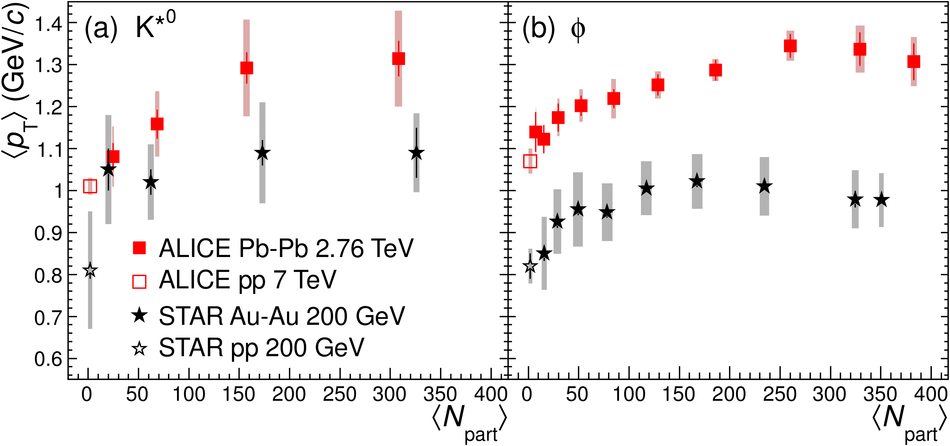## Figure 10

 Mean transverse momentum of $\pi^+$, $\rm K^+$, $\rm K^{*0}$, p, and $\Phi$ in Pb-Pb collisions at $\snn$ = 2.76 TeV (filled symbols) as a function of $\langle N_{part} \rangle$. Also shown are $\langle p_{\rm T} \rangle$ values for the resonances in pp collisions at $\sqrt{s}$ = 7 TeV (open symbols). The measurements for central and mid-central $\Phi$ and p have been shifted horizontally for visibility.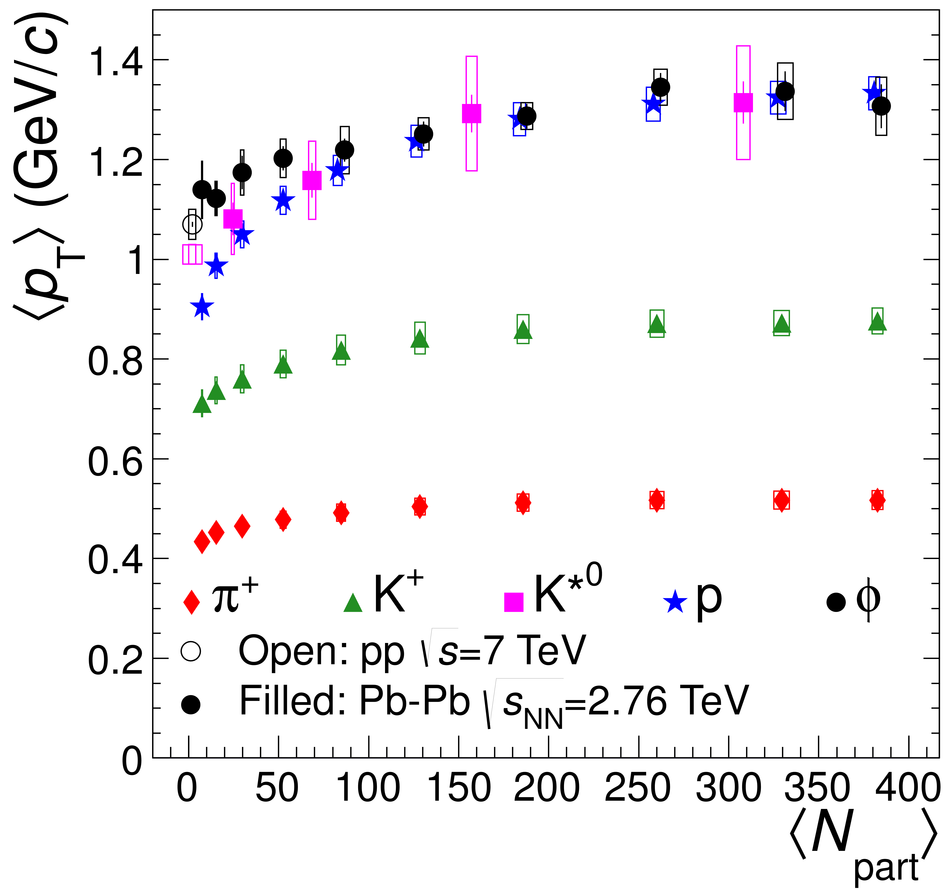## Figure 11

 (a): Comparison of the measured $\Phi$ meson $\pt$ distribution in Pb-Pb collisions at $\snn$ = 2.76 TeV (centrality 0-10\%) to the distributions predicted by the $Krak\acute{o}w$ model, two versions of the VISH2+1 model, the VISHNU model, and the HKM}. The curves show the original predictions, while the horizontal lines show the predicted distributions re-binned so that they have compatible \ptt bins with the measured distribution. (b): The ratio of the re-binned predictions to the measured distribution for $\Phi$ mesons. The shaded band shows the fractional uncertainty of the measured data points.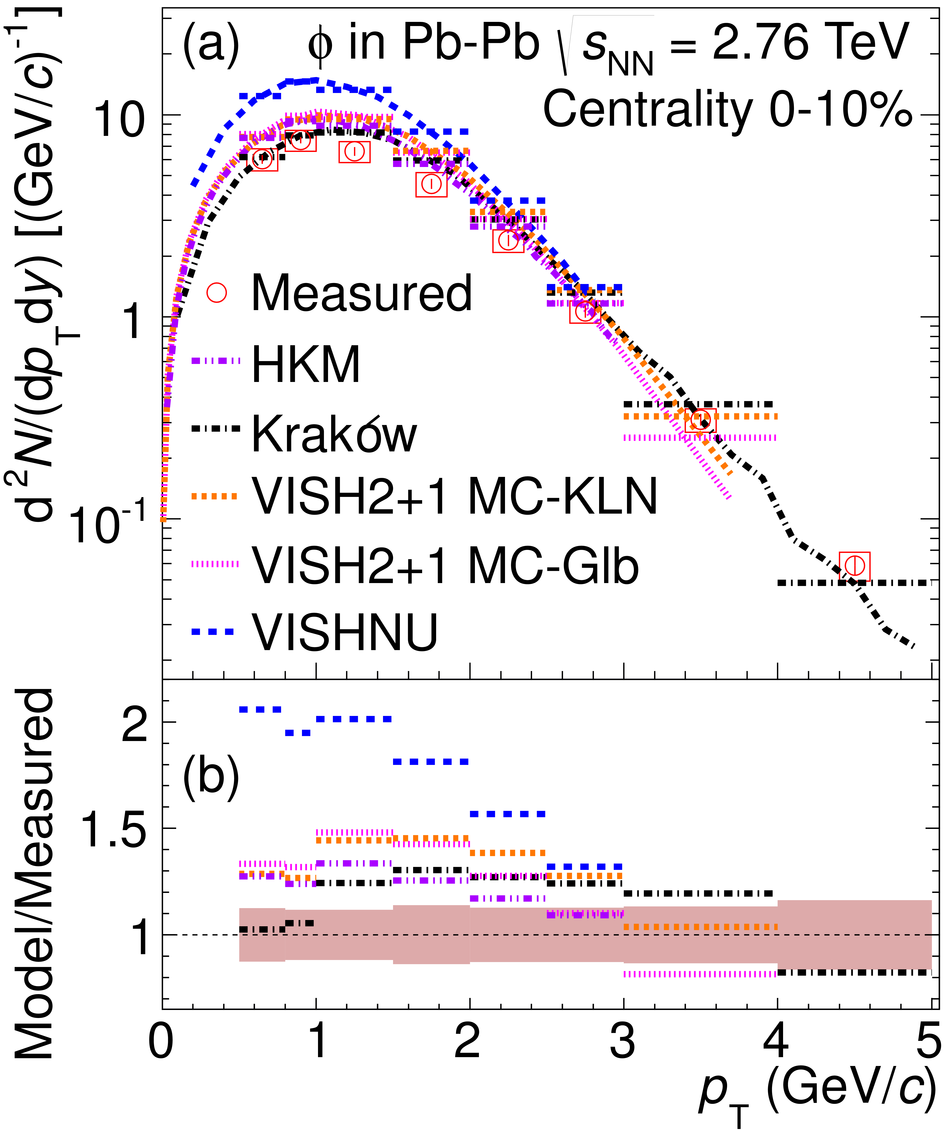## Figure 12

 Ratio $(\Omega^− + \overline{\Omega}^+)/\Phi$ as a function of $\pt$ for Pb-Pb collisions at $\snn$ = 2.76 TeV (centrality 0-10\%), pp collisions at $\sqrt{s}$ = 7 TeV and Au-Au collisions at $\snn$ = 200 GeV (centrality 0-12%). The statistical uncertainties are shown as bars, systematic uncertainties (including $\pt$-uncorrelated and $\pt$-correlated components) are shown as shaded boxes, and the sum in quadrature of the statistical and systematic uncertainties (for the pp data) is shown as open boxes. Also shown are predictions of this ratio made by various models for central Pb-Pb collisions at $\snn$ = 2.76 TeV (centrality 0-20% for HIJING/B$\overline{\mathrm{B}}$, centrality 0-10% for the other models).## Figure 13

 (a): Ratio p/$Phi$ as a function of $\pt$ for Pb-Pb collisions at $\snn$ = 2.76 TeV for four centrality intervals. (b): Ratios of p and $\Phi$ yields to charged pions as a function of $\pt$ for central Pb-Pb collisions at $\snn$ = 2.76 TeV. The p/$\pi$ ratio is presented using two $\pt$ binning schemes: the ratio with its original measured bins is shown along with a recalculated version that uses the same bins as the $\Phi$ meson $\pt$ distribution for $0.5< \pt < 5$ GeV/$c$. In order to show the similarity of the shapes of the two ratios for $\pt < 3$ GeV/$c$, the $\Phi/\pi$ ratio has been scaled so that the $\Phi$ and proton integrated yields are identical. In both panels, the statistical uncertainties are shown as bars and the total systematic uncertainties (including $\pt$-uncorrelated and $\pt$-correlated components) are shown as boxes.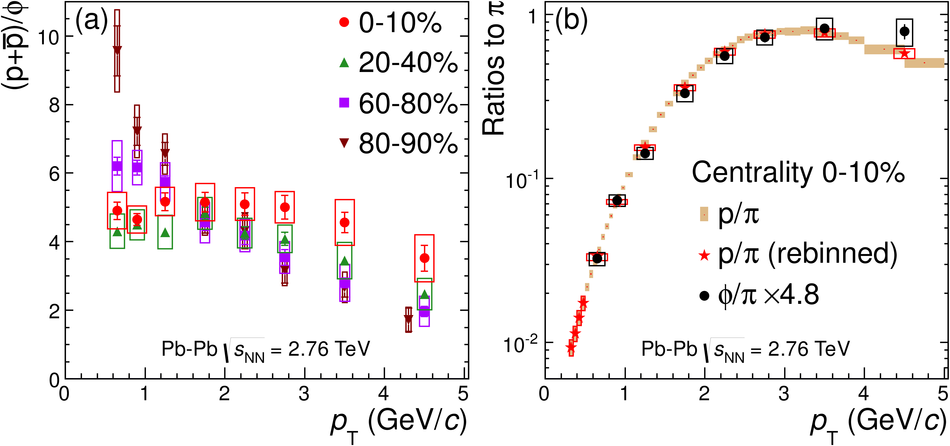## Figure 14

 (a): Enhancement of $\Phi$, $\Lambda$, $\Xi$, and $\Omega$ in Pb-Pb collisions at $\snn$ = 2.76 TeV calculated using pp reference yields (extrapolated for $\Lambda$, interpolated for $\Phi$, $\Xi$, and $\Omega$). Also shown is the enhancement of the $\Phi$ in Au-Au collisions at $\snn$ = 200 GeV, calculated using a measured pp reference yield. The enhancement value reported here is the ratio of the yield (${\rm d} N/\rm d y$) of a particle in A-A collisions to the yield of that particle in pp collisions at the same energy, with both the numerator and denominator normalized by $\langle N_{part} \rangle$. The two most central $\Phi$ enhancement values for Au-Au collisions are for overlapping centrality intervals (0-5\% and 0-10\%). Bars represent the uncertainties in the \ada yields (including centrality-uncorrelated and centrality-correlated components), while the boxes at low values of $\langle N_{part} \rangle$ represent the uncertainties of the pp reference yields. The $\Phi$ and $\Lambda$ measurements at $\langle N_{part} \rangle$=7.5 have been shifted horizontally for visibility. (b): Ratios of particle yields to charged pion yields for Pb-Pb collisions at $\snn$ = 2.76 TeV, Au-Au collisions at $\snn$ = 200 GeV, and pp collisions at $\sqrt{s}$ = 200 GeV, 900 GeV, and 7 TeV. The lines show ratios given by grand-canonical thermal models with temperatures of 170 MeV (upper dashed lines), 164 MeV (solid lines), and 156 MeV (lower dashed lines). The total uncertainties (including centrality-uncorrelated and centrality-correlated components) are shown as bars. Some of the measurements at $\langle N_{part} \rangle$=2 have been shifted horizontally for visibility. The two most central $\Phi/\pi$ values for Au-Au collisions are for overlapping centrality intervals (0-5% and 0-10%).